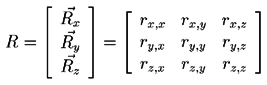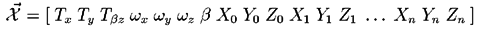Jump back to my NMM index page## Motion (System) Model

The motion models typically used are simplistic and linear. If the camera motion is small relative to the timestep of the estimation process, this is a reasonable assumption. Specifically, we don't model translational velocity or acceleration, only position. The motion is maintained as the global translational and rotational change since the beginning the estimation.

The representation of rotation is slightly more complex. Since the algorithms being considered require the taking the derivative, much of the current literature strongly recommends avoiding the simplest representation, that of Euler angles (rotational angle around the three cartesian coordinate axes.) Instead, a quaternion representation is used. But given the nature of a quaternion representation, which uses four variables to represent a value with three degrees of freedom, direct estimation is not recommended.

The solution is to store the global rotation at a particular time as a quaternion, but to estimate an incremental Euler angle relative to the current rotation. At each step, the global rotation quaternion is updated using the estimate of incremental rotation.

In any case, a rotation matrix R representing the global rotation is generated. The components of the rotation matrix are identified variously as follows :Although some of the examples in literature (Broida90, Kumar89) directly calculated higher derivatives of motion, by including both position and translational velocity, rotation and rotational velocity in the state being estimated, I chose the simpler system model used by Azarbayejani94 (but not his structure representation) The system state vector being estimated is then :The first six parameters represent the camera translation and incremental rotation, the seventh is a camera parameter, and the remainder represent the positions of the feature points in the (arbitrarily scaled) globally frame of reference.

Each feature point is represented by three points, as opposed to the single state variable per feature point proposed by Azarbayejani94. Although a convincing argument is made in their paper that the location of the feature points in the global space is constrained by the measured location in the image plane, plus one "hidden state" variable corresponding to the distance from the image plane, the linearization of the measurement transformation requires the global X and Y location. Calculating these from the one state variable and any one measurement (say the previous one) isn't a good idea due to noisy measurement, requiring that an estimate of the feature points global X and Y location be kept separate from the actual state vector.

Jump back to my NMM index page